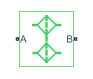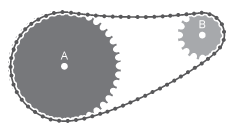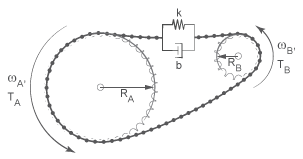# Chain Drive

Power transmission system with chain and two sprockets

•Libraries:
Simscape / Driveline / Couplings & Drives

## Description

The Chain Drive block represents a power transmission system with a chain and two sprockets. The chain meshes with the sprockets, transmitting rotary motion between the two. Power transmission can occur in reverse, that is, from driven to driver sprocket, due to external loads. This condition is known as back-driving.The drive chain is compliant. It can stretch under tension or slacken if loose. The compliance model consists of a linear spring-damper set in a parallel arrangement. The spring resists tensile strain in the chain. The damper resists tensile motion between chain elements.

The spring and damper forces act directly on the sprockets that the chain connects. The spring force is present when one chain branch is taut. The damper force is present continuously. To represent and report a failure condition, the simulation stops and generates an error if the net tensile force in the chain exceeds the specified maximum tension value.

The block accounts for viscous friction at the sprocket joint bearings. During motion, viscous friction causes power transmission losses, reducing chain-drive efficiency. These losses compound due to chain damping. To eliminate power transmission losses in the chain drive, in the Dynamic settings, set the parameters for viscous friction and chain damping to zero.

### Equations

The tensile strain rate in the chain is the difference between the sprocket tangential velocities, which are each the product of the angular velocity and pitch radii. Mathematically,

`$\stackrel{˙}{x}={\omega }_{A}{R}_{A}-{\omega }_{B}{R}_{B},$`

where:

• x is the tensile strain.

• ωA, ωB are the sprocket angular velocities.

• RA, RB are the sprocket pitch radii.

The figure shows the relevant variables.The chain tensile force is the net sum of the spring and damper forces. The spring force is the product of the tensile strain and the spring stiffness constant. This force is zero when the tensile strain is smaller than the chain slack. The damper force is the product of the tensile strain rate and the damping coefficient. Mathematically,

`$F=\left\{\begin{array}{cc}-\left(x-\frac{S}{2}\right)k-\stackrel{˙}{x}b,& x>\frac{S}{2}\\ -\stackrel{˙}{x}b,& \frac{S}{2}\ge x\ge -\frac{S}{2}\\ -\left(x+\frac{S}{2}\right)k-\stackrel{˙}{x}b,& x<-\frac{S}{2}\end{array},$`

where:

• S is the chain slack.

• k is the spring stiffness constant.

• b is the damper coefficient.

The chain exerts a torque on each sprocket equal to the product of the tensile force and the sprocket pitch radius. The two torques act in opposite directions according to these equations:

`${\tau }_{A}=-F·{R}_{A}$`

`${\tau }_{B}=-F·{R}_{B}$`

Where:

• τA is the torque that the chain applies on sprocket A.

• τB is the torque that the chain applies on sprocket B.

In terms of velocity and consideration of friction, these equations apply:

`${\omega }_{A}·{R}_{A}={\omega }_{B}·{R}_{B}$`

`$\left({\tau }_{A}-{\mu }_{A}·{\omega }_{A}\right){R}_{B}=-\left({\tau }_{B}-{\mu }_{B}·{\omega }_{B}\right){R}_{B}$`

Where:

• ωA is the rotational velocity for sprocket A.

• ωB is the rotational velocity for sprocket B.

• μA is the coefficient of viscous friction for sprocket A.

• μB is the coefficient of viscous friction for sprocket B.

### Variables

To set the priority and initial target values for the block variables prior to simulation, use the Initial Targets section in the block dialog box or Property Inspector. For more information, see Set Priority and Initial Target for Block Variables.

Nominal values provide a way to specify the expected magnitude of a variable in a model. Using system scaling based on nominal values increases the simulation robustness. Nominal values can come from different sources, one of which is the Nominal Values section in the block dialog box or Property Inspector. For more information, see Modify Nominal Values for a Block Variable.

## Assumptions and Limitations

• The sprocket tooth ratio equals the sprocket pitch radius ratio.

• Chain inertia is negligible.

## Ports

### Conserving

expand all

Conserving rotational port associated with sprocket A.

Conserving rotational port associated with sprocket B.

## Parameters

expand all

### Geometry

Compliance and backlash model for the block:

• ```Ideal - no chain compliance or backlash``` — Do not model chain stiffness, damping, or backlash.

• ```Model chain compliance and backlash``` — Model chain stiffness, damping, and backlash.

#### Dependencies

If this parameter is set ```Model chain compliance and backlash```, related parameters and variables are enabled.

Radius of the pitch circle for sprocket A. The pitch circle is an imaginary circle passing through the contact point between a chain roller and a sprocket cog at full engagement.

Radius of the pitch circle for sprocket B. The pitch circle is an imaginary circle passing through the contact point between a chain roller and a sprocket cog at full engagement.

Maximum distance the loose branch of the drive chain can move before it is taut. This distance equals the length difference between actual and fully taut drive chains.

If one sprocket is held in place while the top chain branch is taut, then the slack length is the tangential distance that the second sprocket must rotate before the lower chain branch becomes taut.

#### Dependencies

This parameter is enabled when Chain model is set to ```Model chain compliance and backlash```.

### Dynamics

Linear spring constant in the chain compliance model. This constant describes the chain resistance to strain. The spring element accounts for elastic energy storage in the chain due to deformation.

#### Dependencies

This parameter is enabled when Chain model is set to ```Model chain compliance and backlash```.

Linear damping coefficient in the chain compliance model. This coefficient describes the resistance to tensile motion between adjacent elements in the chain. The damper element accounts for power losses in the chain due to deformation.

#### Dependencies

This parameter is enabled when Chain model is set to ```Model chain compliance and backlash```.

Friction coefficient due to the rolling action of the joint bearing for sprocket A in the presence of a viscous lubricant.

Friction coefficient due to the rolling action of the joint bearing for sprocket B in the presence of a viscous lubricant.

### Maximum Tension

These settings are enabled when Chain model is set to ```Model chain compliance and backlash```.

Select whether to constrain the maximum tensile force in the drive chain.

• ```No maximum tension``` — Chain tension can be arbitrarily large during simulation.

• ```Specify maximum tension``` — Chain tension must remain lower than a maximum value. If the tension exceeds this value, the simulation generates an error and stops.

#### Dependencies

When this parameter is set to ```Specify maximum tension```, related parameters are enabled.

This parameter is enabled when Chain model is set to ```Model chain compliance and backlash```.

Maximum allowed value of the tensile force acting in the chain.

#### Dependencies

This parameter is enabled when both of these conditions are met:

• In the Geometry settings, the Chain model parameter is set to ```Model chain compliance and backlash```.

• Im the Maximum tension settings, the Maximum tension parameter is set to ```Specify maximum tension```.

## Version History

Introduced in R2012a